StatLect

# Model selection criteria

Model selection criteria are rules used to select the best statistical model among a set of candidate models.

In this lecture we focus on criteria used to select models that have been estimated by the maximum likelihood method.

For criteria used to select linear regression models, go to this lecture.## How the criteria are used

We will carefully explain the theory behind model selection criteria. But before doing that, let us preview how model selection criteria work:

1. we define a set of candidate models;

2. we estimate the parameters of each model by maximum likelihood;

3. we use the estimated parameters to compute the log-likelihood of each model;

4. we assign a score to each model; the score has the following characteristics:

1. it is decreasing in the log-likelihood (the better the model fits the data, the lower the score);

2. it is increasing in the number of parameters (the more complex the model is, the higher the score);

5. we choose the model that has the lowest score.

## Preview of popular criteria

Let us see how model scores are calculated, according to some popular criteria.

Denote by:

•the number of parameters;

•the sample size;

•the log-likelihood.

Model scores are calculated as follows:

1. Akaike Information Criterion (AIC):2. Corrected Akaike Information Criterion (AICc):3. Hannan-Quinn Information Criterion (HQIC):4. Bayesian Information Criterion (BIC):## Differences and commonalities

All of these criteria generate a trade-off between goodness of fit (measured by the log-likelihood) and model complexity (the number of parameters).

The trade-off discourages overfitting.

However, some of these criteria impose a stronger penalty for model complexity.

In the list above, the criteria are ordered based on the strength of the penalty: the AIC imposes the mildest penalty, while the BIC has the strongest one.

## Statistical models

We now explain the theory behind selection criteria in more detail.

First of all, we need to define precisely what we mean by statistical model.

A statistical model is a set of probability distributions that could have generated the data we are analyzing.

## Examples of statistical models

Suppose that we observedata pointsindependently drawn from the same probability distribution (in technical terms, they are IID draws).

### A normal model

If we assume that the draws come from a normal distribution, then we are formulating a statistical model: we are restricting our attention to the set of all normal distributions and we are ruling out all the probability distributions that are not normal.

The normal distribution has two parameters, the meanand the variance.

So, the set of distributions we are considering (the statistical model) includes many normal distributions: one for each possible couple.

### An exponential model

If instead we assume that the data have been drawn from an exponential distribution, then we are formulating an alternative model.

The exponential distribution has one parameter, called rate parameter.

Our statistical model is a set including many possible distributions: one for each possible value of the parameter.

### The selection

This example, although admittedly unrealistic, introduces in a simple manner the problem that we are going to deal with: how do we select one model (normal vs exponential distribution in the example) if we deem that two or more alternative models are plausible?

## Notation and main assumptions

Let us denote the vector of observed data by.

We assume that the data are continuous.

Therefore, a model foris a family of joint probability density functionsparametrized by a parameter vectorfor each model.

We focus on continuous distributions in order to simplify the discussion, but everything we say is valid also for discrete distributions, with straightforward modifications (replace probability densities with probability mass functions).

We denote bythe unknown probability distribution that generated the data.

Finally,is the index of the model selected by a model selection criterion. Clearly,can range betweenand.

## Example - Continued

In the example above the vectorcontains thedata points:The number of models is.

### Parameters

The two parameter vectors arefor the normal distribution andfor the exponential distribution.

### Densities

The joint probability density function for the first model is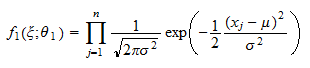because the joint density of a vector of independent random variables is equal to the product of their marginal densities.

The joint probability density function for the second model is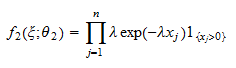whereis an indicator function (equal to 1 ifand to 0 otherwise).

## Maximum likelihood estimation

We assume that model parameters are estimated by maximum likelihood (ML).

We denote bythe ML estimates of the parameters of themodels.

If you want to see some examples of how ML estimates are derived, you can have a look at these two lectures:

## The general criterion

Akaike (1973) was the first to propose a general criterion for selecting models estimated by maximum likelihood.

He proposed to minimize the expected dissimilarity between the chosen modelat the ML estimate and the true distribution.

### Measuring the expected dissimilarity

The dissimilarity between an estimated model and the true distribution is measured by the Kullback-Leibler divergence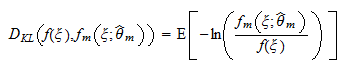where the expected value is with respect to the true densityThe expected dissimilarity is computed aswhere the expectation is over the sampling distribution of, which, being a function of the sample, is regarded as stochastic.

### Approximating the expected dissimilarity

Ideally, we would like to select the model that minimizes the expected dissimilarity: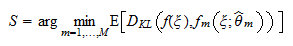However, the expected dissimilarity cannot be computed exactly because the true distributionand the sampling distribution ofare unknown.

Akaike (1973) proposed an approximation to the expected dissimilarity that can be easily computed, giving rise to the so-called Akaike Information Criterion (AIC).

As proved, for example, by Burnham and Anderson (2004), other popular selection criteria such as the AIC corrected for small-sample bias (AICc; Sugiura 1978, Hurvich and Tsai 1989) and the Bayesian Information Criterion (BIC; Schwarz 1978) are based on different approximations of the same measure of expected dissimilarity.

## Popular criteria

We briefly present here the most popular selection criteria.

### Akaike Information Criterion (AIC)

According to the Akaike Information Criterion, the selected modelsolves the minimization problemwhere the value of the-th model is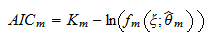whereis the number of parameters to be estimated in the-th model.

Note that any linear transformation applied to all model values does not change the selected model. As a matter of fact, many references define the value of the-th model as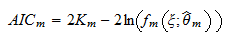### Corrected Akaike Information Criterion (AICc)

An approximation that is more precise in small samples is the so-called corrected Akaike Information Criterion (AICc), according to which the value to be minimized is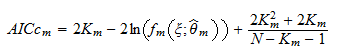whereis the size of the sample being used for estimation.

### Bayesian Information Criterion (BIC)

Another popular criterion is the Bayesian Information Criterion, according to which the selected model is the one that achieves the minimum value of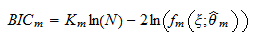Its name is due to the fact that it can be justified by using some Bayesian arguments.

If the sample sizeis large, it penalizes model complexity much more than Akaike's criteria.

### Hannan-Quinn Information Criterion (HQIC)

A compromise between Akaike's criteria (mild penalty for the number of parameters) and the Bayesian criterion (large penalty) is provided by the Hannan-Quinn Information Criterion: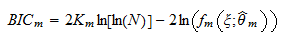## The penalty for complexity

As you might have noticed, all of these criteria penalize the dimension of the model: the higher the number of parametersis, the more modelis penalized.

This penalty for complexity is typical of model selection criteria: a model with many parameters is more likely to over-fit, that is, to have a spuriously high value of the log-likelihood.

For a discussion of over-fitting see the lecture on the R squared of a linear regression.

The complexity penalty is also related to the so-called bias-variance trade-off: by increasing model complexity, we usually decrease the bias and increase the variance.

Beyond a certain degree of complexity, increases in variance are larger than reductions in bias, and, as a consequence, the quality of our inferences becomes worse.

## References

Akaike, H., 1973. Information theory as an extension of the maximum likelihood principle. In: Petrov, BN and Csaki, F. In Second International Symposium on Information Theory. Akademiai Kiado, Budapest, pp. 276-281.

Burnham, K.P. and Anderson, D.R., 2004. Multimodel inference: understanding AIC and BIC in model selection. Sociological methods & research, 33(2), pp. 261-304.

Hurvich, C.M. and Tsai, C.L., 1989. Regression and time series model selection in small samples. Biometrika, 76(2), pp. 297-307.

Schwarz, G., 1978. Estimating the dimension of a model. The annals of statistics, 6(2), pp. 461-464.

Sugiura, N., 1978. Further analysis of the data by Akaike's information criterion and the finite corrections, in Statistics Theory and Methods, 7(1), pp. 13-26.